NetworkX

#### Previous topic

weighted_projected_graph

#### Next topic

overlap_weighted_projected_graph

# collaboration_weighted_projected_graph¶

collaboration_weighted_projected_graph(B, nodes)[source]

Newman’s weighted projection of B onto one of its node sets.

The collaboration weighted projection is the projection of the bipartite network B onto the specified nodes with weights assigned using Newman’s collaboration model [R123]: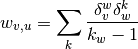where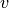and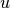are nodes from the same bipartite node set, and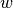is a node of the opposite node set. The value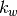is the degree of nodein the bipartite network and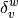is 1 if nodeis linked to nodein the original bipartite graph or 0 otherwise.

The nodes retain their attributes and are connected in the resulting graph if have an edge to a common node in the original bipartite graph.

Parameters : B : NetworkX graph The input graph should be bipartite. nodes : list or iterable Nodes to project onto (the “bottom” nodes). Graph : NetworkX graph A graph that is the projection onto the given nodes.

Notes

No attempt is made to verify that the input graph B is bipartite. The graph and node properties are (shallow) copied to the projected graph.

References

 [R123] (1, 2) Scientific collaboration networks: II. Shortest paths, weighted networks, and centrality, M. E. J. Newman, Phys. Rev. E 64, 016132 (2001).

Examples

>>> from networkx.algorithms import bipartite
>>> B = nx.path_graph(5)